## Chapter 3 Pair of Linear Equations in Two Variables R.D. Sharma Solutions for Class 10th Math Exercise 3.1

Exercise 3.1

1. Akhila went to a fair in her village. She wanted to enjoy rides in the Giant Wheel and play Hoopla (a game in which you throw a rig on the items kept in the stall, and if the ring covers any object completely you get it.) The number of times she played Hoopla is half the number of rides she had on the Giant Wheel. Each ride costs Rs 3, and a game of Hoopla costs Rs 4. If she spent Rs 20 in the fair, represent this situation algebraically and graphically.

Solution

The pair of equations is formed :Let us represent these equations graphically. For this, we need at least two solutions for
each equation. We give these solutions in Table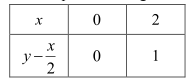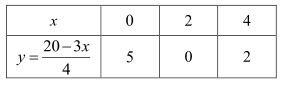Recall from Class IX that there are infinitely many solutions of each linear equation. So
each of you choose any two values, which may not be the ones we have chosen. Can you
guess why we have chosen  x = 0  in the first equation and in the second equation? When
one of the variables is zero, the equation reduces to a linear equation is one variable, which
can be solved easily. For instance, putting  x = 0 in Equation (2), we get 4y = 20 i.e,
y = 5.
Similarly , putting y = 0 in Equation(2), we get 3x = 20 i.e., x = 20/3 . But as 20/3 is
not an integer, it will not be easy to plot exactly on the graph paper. So, we choose y = 2 which gives x = 4, an integral value.Plot the points A(O,O) B(2,1) and P(O,5), Q(412), corresponding to the draw the lines AB and PQ, representing the equation x-2y = 0 and 3x+4y = 20, as shown in figure
In fig., observe that the two lines representing the two equations are intersecting at the point(4,2),

2. Aftab tells his daughter, "Seven years ago, I was seven times as old as you were then. Also, three years from now, I shall be three times as old as you will be". Is not this interesting? Represent this situation algebraically and graphically.

Solution

Let the present age of Aftab and his daughter be x and y respectively. Seven years ago.
Age of Ahab = x - 7
Age of his daughter = y - 7
According to the given condition.
(x-7) = 7 (y-7)
⇒ x-7 = 7y - 49
⇒ x-7 y = -42
Three years hence
Age of Aftab = x+3
Age of his daughter = y+3
According to the given condition,
(x+3) = 3 (x+3)
⇒ x+3 = 3y + 9
⇒ x - 3y = 6
Thus, the given condition can be algebraically represented as
x-7y = -42
x - 3y = 6
x-7y = -42 ⇒ x = -42 + 7y

Three solution of this equation can be written in a table as follows:x -3y = 6 ⇒ x = 6 + 3y

Three solution of this equation can be written in a table as follows:The graphical representation is as follows:Concept insight: In order to represent a given situation mathematically, first see what we need to find out in the problem. Here. Aftab and his daughters present age needs to be found so, so the ages will be represented by variables z and y. The problem talks about their ages seven years ago and three years from now. Here, the words ’seven years ago’ means we have to subtract 7 from their present ages. and ‘three years from now’ or three years hence means we have to add 3 to their present ages. Remember in order to represent the algebraic equations graphically the solution set of equations must be taken as whole numbers only for the accuracy. Graph of the two linear equations will be represented by a straight line.

3. The path of a train A is given by the equation 3x+4y-12=0 and the path of another train B is given by the equation 6x+8y-48=0. Represent this situation graphically .

Solution

The paths of two trains are giver by the following pair of linear equations.
3x+4y-12=0 ...(1)
6x+8y-48=0 ...(2)

In order to represent the above pair of linear equations graphically. We need two points on
the line representing each equation. That is, we find two solutions of each equation as given
below:
We have,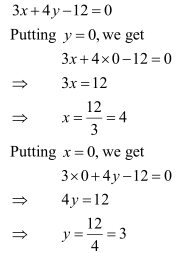Thus, two solution of equation 3x+4y-12=0 are (0,3) and (4,0)
We have ,
6x+8y-48=0
Putting x = 0, we get
6×0+8y-48=0
⇒ 8y= 48Thus, two solution of equation 6x+8y-48=0 are (0,6) and (8,0)Clearly, two lines intersect at (-1,2)
Hence, x = -1, y=2 is the solution of the given system of equations .

4. Gloria is walking along the path joining (− 2, 3) and (2, − 2), while Suresh is walking along the path joining (0, 5) and (4, 0). Represent this situation graphically.

Solution

It is given that Gloria is walking along the path joining (-2,3) and (2,-2), while Suresh is walking along the path joining (0,5) and (4,0).We observe that the lines are parallel and they do not intersect anywhere.

5. On comparing the ratios a1/a2 , and c1/c2 and without drawing them, find out whether the lines representing the following pairs of linear equations intersect at a point, are parallel or coincide:
(i) 5x-4y+8 = 0, 7x+6y-9 = 0
(ii) 9x+3y+12 = 0, 18x+6y+24 = 0,
(iii) 6x-3y+10 = 0, 2x-y+9 = 0

Solution

(i) We have,
5x-4y+8=0
7x+6y-9=0
Here,
a1=5,b1=-4,c1=8
a2=7,b2=6,c2=-9
We have,∴ Two lines are intersecting with each other at a point.

(ii) 9x+3y+12=0
18+6y+24=0
Here
a1=9,b1=3,c1=12
a2=18,b2=6,c2=24

Now,∴ Both the lines coincide .

(iii) 6x-3y+10=0
2x-y+9=0
Here,
a1=6, b1=-3, c1=10
a2=2, b2=-1, c2=9
Now,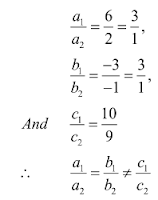The lines are parallel

6. Given the linear equation 2x + 3y - 8 = 0, write another linear equation in two variables such
that the geometrical representation of the pair so formed is:
(i) intersecting lines (ii) parallel lines (iii) coincident lines.

Solution

We have ,
2x+3y-8=0
Let another equation of line is :
4x+9y-4=0
Here,
a1=2,b1=3,c1=-8
a2=4,b2=9,c2=-4
Now,∴ 2x+3y-8=0 and 4x+9y-4=0 intersect each other at one point.
Hence, required equation of line is 4x+9y-4=0

(ii) We have,
2x+3y-8=0
Let another equation of line is:
4x+6y-4=0
Here,
a1=2,b1=3,c1=-8
a2=4,b2=6,c2=-4
Now,∴ Lines are parallel to each other.
Hence, required equation of line is  4x+6y-4=0.

7. The cost of 2kg of apples and 1 kg of grapes on a day was found to be Rs 160. After amonth, the cost of 4kg of apples and 2kg of grapes is Rs 300. Represent the situation algebraically and geometrically.

Solution

Let the cost of 1 kg of apples and 1 kg grapes be Rs x and Rs y.
The given conditions can be algebraically represented as
2x+y=160
4x+2y=300
2x+y=160⇒y=160-2x
Three solutions of this equation can be written in a table as follows: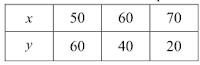4x+2y=300⇒y=300-4x/2
Three solutions of this equation can be written in a table as follows: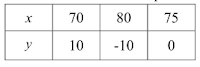The graphical representation is as follows:Concept insight: cost of apples and grapes needs to be found so the cost of 1 kg apples and 1kg grapes will be taken as the variables from the given condition of collective cost of apples and grapes, a pair of linear equations in two variables will be obtained. Then In order to represent the obtained equations graphically, take the values of variables as whole numbers only. Since these values are Large so take the suitable scale.